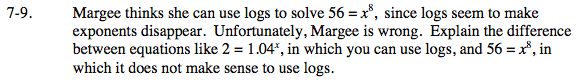### Home > INT3 > Chapter 7 > Lesson 7.1.1 > Problem7-9

7-9.

Margee thinks she can use logs to solve 56 = x8, since logs seem to make exponents disappear. Unfortunately, Margee is wrong. Explain the difference between equations like 2 = 1.04x, in which you can use logs, and 56 = x8, in which it does not make sense to use logs. Homework Help ✎Look at where the variable is in each equation.

Logarithms are used to solve equations where the variable is the exponent.
Roots (or raising to a fractional exponent) are used to solve equations where the exponent is known and the variable is the base.